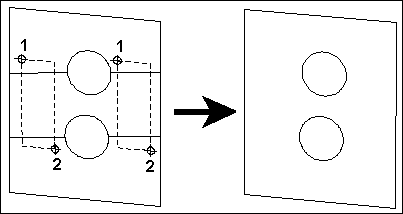# Hidden Edge by Section

Erases cut-plane lines which are sometimes a result of the Cut Plane, Polygon Subtract, and Solid Subtract commands.

After executing a Cut Plane, Polygon Subtract, or Solid Subtract command, select the Hidden Edge by Section command. Set two points to define a bounding box that encloses or touches the cut-plane lines, so that they will be hidden from the view or from a printout of the drawing.

Example: Draw a large square plane and two small circular planes. Draw the circular planes so that they are contained by the square plane with respect to the XY plane. Use Polygon Subtract to subtract the circular planes from the square plane. Notice the cut-plane lines which are a necessity of this kind of operation. Choose the

Hidden Edge by Section command and set a point at one corner of the area that contains the cut-plane lines to the left of the circular holes. This point also defines one of the corners for a bounding (or rubber-band) box that moves with the cursor. Move the cursor so that the bounding box touches both of the edges to the left of the holes. Set another point. Both of the cut-plane lines to the left of the holes will be removed from the view and will not be printed in subsequent printouts. Now do the cut-plane lines on the right side of the holes.